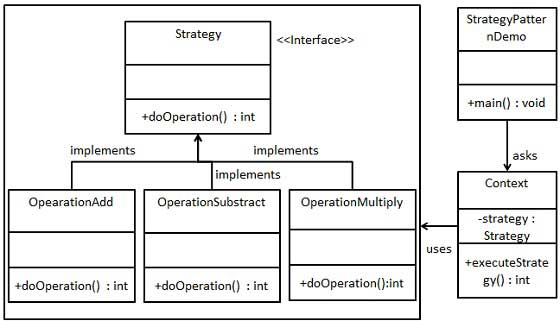# 策略模式

## 实现

StrategyPatternDemo，我们的演示类使用 Context 和策略对象来演示 Context 在它所配置或使用的策略改变时的行为变化。### 步骤 1

Strategy.java

```public interface Strategy {
public int doOperation(int num1, int num2);
}
```

### 步骤 2

```public class OperationAdd implements Strategy{
@Override
public int doOperation(int num1, int num2) {
return num1 + num2;
}
}
```

OperationSubstract.java

```public class OperationSubstract implements Strategy{
@Override
public int doOperation(int num1, int num2) {
return num1 - num2;
}
}
```

OperationMultiply.java

```public class OperationMultiply implements Strategy{
@Override
public int doOperation(int num1, int num2) {
return num1 * num2;
}
}
```

### 步骤 3

Context.java

```public class Context {
private Strategy strategy;

public Context(Strategy strategy){
this.strategy = strategy;
}

public int executeStrategy(int num1, int num2){
return strategy.doOperation(num1, num2);
}
}
```

### 步骤 4

StatePatternDemo.java

```public class StrategyPatternDemo {
public static void main(String[] args) {
Context context = new Context(new OperationAdd());
System.out.println("10 + 5 = " + context.executeStrategy(10, 5));

context = new Context(new OperationSubstract());
System.out.println("10 - 5 = " + context.executeStrategy(10, 5));

context = new Context(new OperationMultiply());
System.out.println("10 * 5 = " + context.executeStrategy(10, 5));
}
}
```

### 步骤 5

```10 + 5 = 15
10 - 5 = 5
10 * 5 = 50
```

App下载